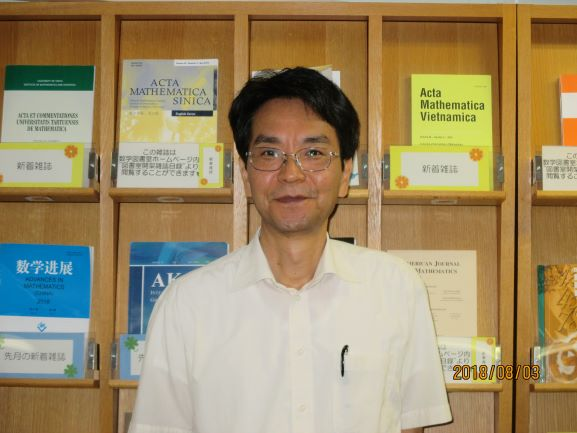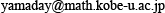Yasuhiko YAMADA Yasuhiko YAMADA Department of Mathematics, Graduate School of Science, Kobe University Division : Analysis Professor Building B, Room 308Personal Website

 Research Field : Integrable systems: Special functions: Mathematical Physics Research Summary : The traditional term "integrable system" means a mathematical model that admits exact solutions. In many cases, a beautiful mathematical structure lies in such models. I am studying them from various points of view such as discrete, continuous, classical and quantum. Currently, I am working on the quantization of the iso-monodromy equations, their relation to conformal field theories and gauge theories. Primary Publications : A.Tsuchiya, K.Ueno and Y.Yamada, "Conformal field theory on universal family of stable curves with gauge symmetries", Adv. Stud. Pure Math. 19, (1989) 459--566. H.Awata, A.Tsuchiya and Y.Yamada, "Integral formulas for the WZNW correlation functions", Nucl. Phys. B 365 (1991), no. 3, 680--696. T.Kawai, Y.Yamada and S.-K.Yang, "Elliptic genera and $N=2$ superconformal field theory", Nuclear Phys. B 414 (1994), no. 1-2, 191--212. T.Eguchi, Y.Yamada and S.-K.Yang, "On the Genus Expansion in the Topological String Theory", Reviews in Mathematical Physics 7 (1995) 279--310. A.Nakayashiki and Y.Yamada, "Kostka polynomials and Energy functions in Solvable lattise models", Selecta Math. 3 (1997) 547--599. M.Noumi and Y.Yamada, "Affine Weyl groups, discrete dynamical systems and Painlevé equations", Comm. Math. Phys. 199 (1998) 281--295. G.Hatayama, A.Kuniba, M.Okado, T.Takagi and Y.Yamada, "Remarks on fermionic formula," Contemp. Math., 248, Amer. Math. Soc., Providence, RI, (1999) 243--291. K.Kajiwara, M.Noumi and Y.Yamada, "Discrete dynamical systems with $W(A^{(1)}_{m-1} \times A^{(1)}_{n-1})$ symmetry", Lett. Math. Phys. 60 (2002), no. 3, 211--219. K.Kajiwara, T.Masuda, M.Noumi, Y.Ohta, Y.Yamada, "${}_{10}E_9$ solution to the elliptic Painlevé equation", J. Phys. A 36 (2003), no. 17, L263--L272.